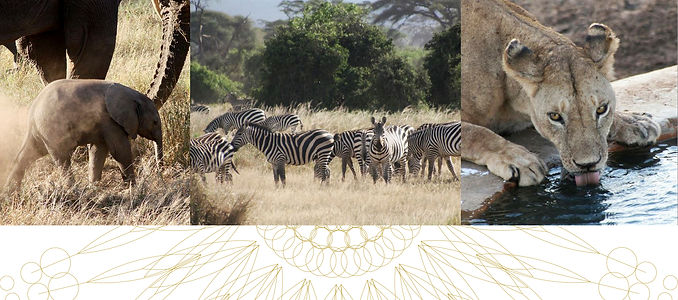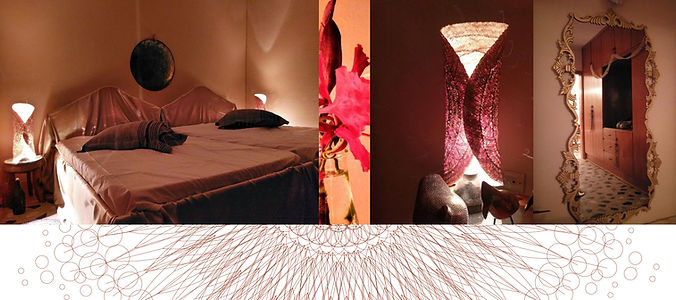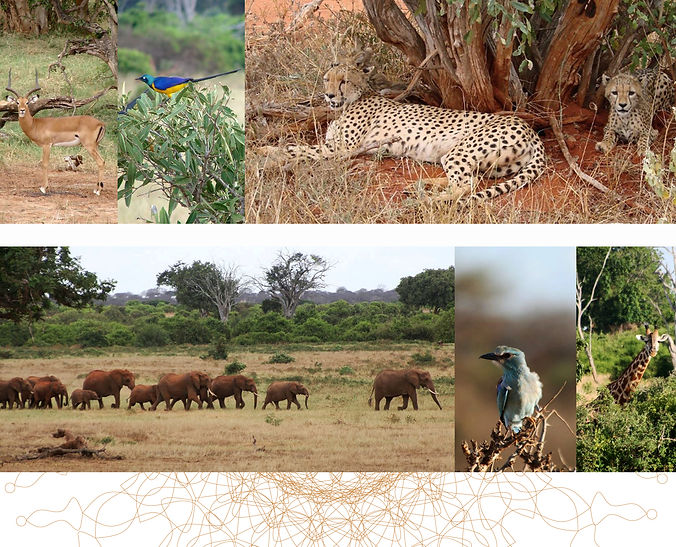top of page## Rewards

##### Make a pledge without a reward

Pledge any amount

#### Pledge €139 or more

###### ​

|       ).(  .  .  ).(      ).(  .  .  ).(  .  .  ).(      ||       Y  O  U  R      R  E  W  A  R  D  S      |#### Pledge €219 or more

###### |       ).(  .  .  ).(      ).(  .  .  ).(  .  .  ).(      ||       Y  O  U  R      R  E  W  A  R  D  S      |#### Pledge €149 or more

###### |       ).(  .  .  ).(      ).(  .  .  ).(  .  .  ).(      ||       Y  O  U  R      R  E  W  A  R  D  S      |#### Pledge €199 or more

###### |       ).(  .  .  ).(      ).(  .  .  ).(  .  .  ).(      ||       Y  O  U  R      R  E  W  A  R  D  S      |#### Pledge €199 or more

###### |       ).(  .  .  ).(      ).(  .  .  ).(  .  .  ).(      ||       Y  O  U  R      R  E  W  A  R  D  S      |#### Pledge €249 or more

###### |       ).(  .  .  ).(      ).(  .  .  ).(  .  .  ).(      ||       Y  O  U  R      R  E  W  A  R  D  S      |#### Pledge €249 or more

###### |       ).(  .  .  ).(      ).(  .  .  ).(  .  .  ).(      ||       Y  O  U  R      R  E  W  A  R  D  S      |#### Pledge €249 or more

###### |       ).(  .  .  ).(      ).(  .  .  ).(  .  .  ).(      ||       Y  O  U  R      R  E  W  A  R  D  S      |#### Pledge €249 or more

###### |       ).(  .  .  ).(      ).(  .  .  ).(  .  .  ).(      ||       Y  O  U  R      R  E  W  A  R  D  S      |#### Pledge €249 or more

###### |       ).(  .  .  ).(      ).(  .  .  ).(  .  .  ).(      ||       Y  O  U  R      R  E  W  A  R  D  S      |#### Pledge €299 or more

###### |       ).(  .  .  ).(      ).(  .  .  ).(  .  .  ).(      ||       Y  O  U  R      R  E  W  A  R  D  S      |#### Pledge €449 or more

###### |       ).(  .  .  ).(      ).(  .  .  ).(  .  .  ).(      ||       Y  O  U  R      R  E  W  A  R  D  S      |#### Pledge €499 or more

###### |       ).(  .  .  ).(      ).(  .  .  ).(  .  .  ).(      ||       Y  O  U  R      R  E  W  A  R  D  S      |#### ​

###### |       ).(  .  .  ).(      ).(  .  .  ).(  .  .  ).(      ||       Y  O  U  R      R  E  W  A  R  D  S      |#### Pledge €999 or more

###### |       ).(  .  .  ).(      ).(  .  .  ).(  .  .  ).(      ||       Y  O  U  R      R  E  W  A  R  D  S      |#### Pledge €999 or more

###### |       ).(  .  .  ).(      ).(  .  .  ).(  .  .  ).(      ||       Y  O  U  R      R  E  W  A  R  D  S      |#### Pledge €1,499 or more

###### |       ).(  .  .  ).(      ).(  .  .  ).(  .  .  ).(      ||       Y  O  U  R      R  E  W  A  R  D  S      |#### Pledge €1,999 or more

###### |       ).(  .  .  ).(      ).(  .  .  ).(  .  .  ).(      ||       Y  O  U  R      R  E  W  A  R  D  S      |#### Pledge €7,777 or more

###### |       ).(  .  .  ).(      ).(  .  .  ).(  .  .  ).(      ||       Y  O  U  R      R  E  W  A  R  D  S      |Awards 1
Anchor 1a
Anchor 2
Anchor 2a
Anchor 3
Anchor 4
Anchor 5
Anchor 6
Anchor 7
Anchor 8
Anchor 9
Anchor 10
Anchor 11
Anchor 12
Anchor 13
Anchor 14
Anchor 15
Anchor 16
Anchor 17
Anchor 18
Anchor 19
bottom of page